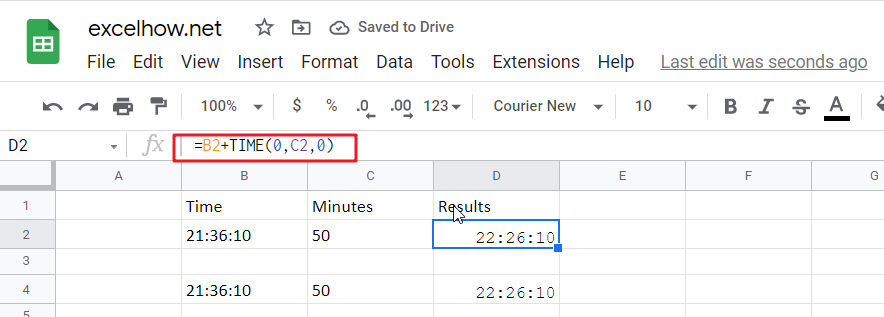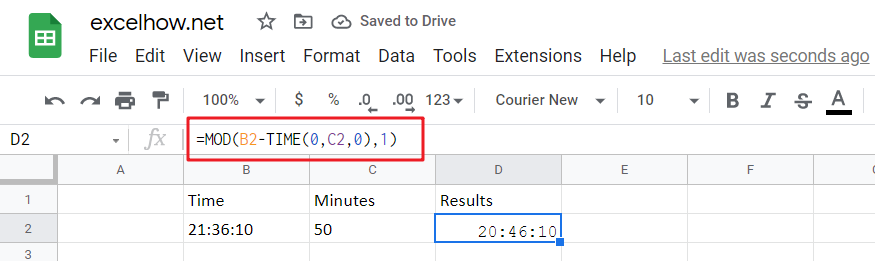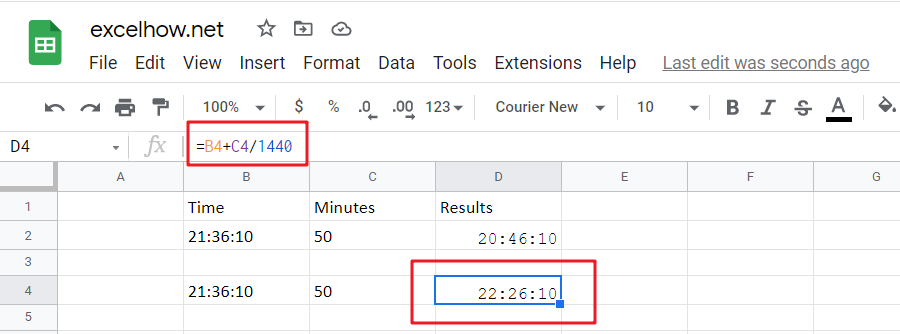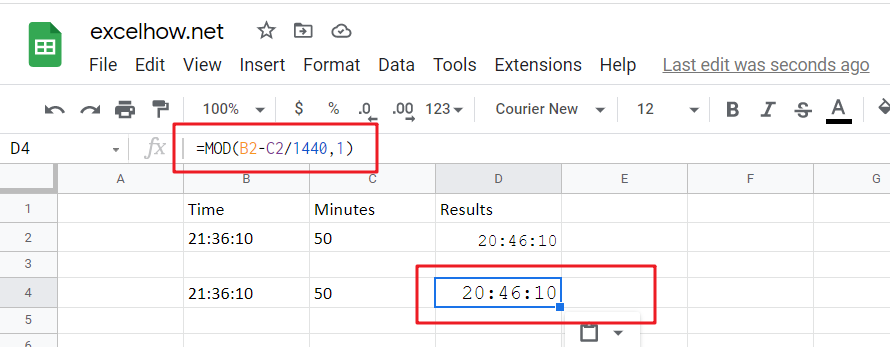Adding whole hours or decimal minutes to a given time in google sheets is a fairly simple process. You can do this by using the Start_time+TIME(0,minutes,0) and Start_time+minutes/1440 functions. In this add minutes to time guide, we will show you how to do this.If you want to add whole minutes to a given time, you can use the following formula:

`=Start_time+TIME(0, minutes,0)`
• Start_time: the time that you want to add minutes to
• Minutes: the minutes that you wish to add

This function returns a time formatted string.

Cell C2 shows the number of minutes you want to add, while Cell D2 displays a formula that will calculate how many more cells need changing.

`=B2+TIME(0,C2,0)`

Or

`=B4+C4/1440`

To see the result, press Enter key.### Explanation

The TIME function is a great way to convert decimal numbers into an appropriate time format. Here, we change the number of minutes from 50 (which converts it into PM) and then add:00 before adding another set amount for minutes-in, in this case, 0 because there aren’t any other digits available on our keyboard!

Note: To subtract the minutes from a time period, you need to use this formula.

`= MOD(Start_time-TIME(0, minutes,0),1)`

For Instance, Cell B2 shows time, and cell C2 shows the number of minutes you want to subtract. If you want to subtract a number of minutes, use the below formula:

`=MOD(B2-TIME(0,C2,0),1)`

To see the result, hit Enter key.The MOD (modulus) function is an important tool for financial professionals. It can be used to flip negative numbers into positive ones yield accurate results in most cases!

## Add decimal Minutes to time

Generic formula:

`=Start_time+ minutes /1440`
• Start_time: the time that you wish to add minutes to.
• minutes: the minutes that you wish to add to start_time.

The value should be returned in time format.

For instance, cell B2 contains the time value, and cell C2 displays the number of minutes you want to add. Please see the below formula for this purpose.

`=B2+C2/1440`

To see the result hit Enter key.24 hours in a day, and one minutes is 1/1440th of the whole duration.

Note: Subtracting the minutes from time is easy with this formula.

`=MOD(Start_time- minutes /1440,1)`

The number of minutes you want to subtract is displayed in cell C2, and time is displayed in B2. To find the correct formula, please use this simple equation:

`=MOD(B2-C2/1440,1)`

To see the result hit Enter key.When google sheets detects negative values in a cell, it converts them to positive with the MOD function. This means you can just flip your negatives around and get an accurate time reflecting how long something takes!

## Related Functions

The Google Sheets TIME function returns a date in serial number format for a particular time (the hour, minute and second).The syntax of the TIME function is as below:= TIME(hour, minute, second)…
the Google Sheets MOD function returns the remainder of two numbers after division. So you can use the MOD function to get the remainder after a number is divided by a divisor in Excel. The syntax of the MOD function is as below:=MOD (number, divisor)….
Related Posts

Find the Closest Data to the Data Provided in Google Sheets

In our daily work, we may encounter such an issue that to find the closest value to a certain value. In fact, google sheets internal functions can help us solve this problem. In today’s article, we will show you how ...

Basic Array Formula With Examples in Google Sheets

Are you searching for an article for getting the basic array formula with different examples for better understanding? Then congratulations because you have just landed on the right article. In this article, you would get to know the basic array ...

Average Of Numbers With Multiple Criteria in Google Sheets

Have you ever come across a task to calculate the average of the numbers with respect to multiple criteria in google sheets? Are you tired of doing this cumbersome task manually? Are you willing to do this task smartly in ...

Calculate Average Of Last 5 Or N Values In Columns in Google Sheets

Suppose you come across a task where you need to calculate the average of the last 2 or 3 numeric values in google sheets, then what would you do? If you are new to google sheets, then your first attempt ...

Find And Retrieve Missing Values in Google Sheets

Find and retrieve is a common basic operation in Google Sheets for tables. In out daily work, we may encounter this situation that some data was lost after several operations on a table in Google Sheets. In fact, we can ...

Calculating Average Of The Numbers in Google Sheets

Are you weary of investing a lot of time and effort in manually calculating the average of the numbers by including or excluding 0 and calculating the average of the top 3 scores? Then congratulations because you have just landed ...

Calculate The Average Of The Last 3, 5, Or N Numeric Values In Google Sheets

As an Google sheets user, you might have come across a task in which you need to calculate the average values of the last 2 numeric values, and you might have done this task manually but suppose if the last ...